# Catch up on the latest AI articles# Here Comes AugMax, A Data Extension Method That Overcomes Model Weaknesses!

3 main points
✔️ Propose AugMax, a new data augmentation strategy that significantly improves robustness by strategically increasing data diversity and difficulty
✔️ AugMAX combines multiple transformed images that have undergone data augmentation with convex merging to maintain diversity but not deviate too much from the original image. AugMix and hostile perturbation are combined to improve data diversity and difficulty.
✔️ Performance against CIFAR-10-C, CIFAR100-C, ImageNet-C, and Tiny ImageNet is verified using three metrics and confirmed to outperform state-of-the-art performance.

written by Haotao WangChaowei XiaoJean KossaifiZhiding YuAnima AnandkumarZhangyang Wang
(Submitted on 26 Oct 2021 (v1), last revised 1 Jan 2022 (this version, v3))

Subjects: Computer Vision and Pattern Recognition (cs.CV); Machine Learning (cs.LG)code：The images used in this article are from the paper, the introductory slides, or were created based on them.

## Introduction

Since deep learning methods have demonstrated high performance in image recognition, many models using convolutional neural networks have been proposed for image classification. Many of these models can achieve high accuracy if the distribution of training data and that of test data are identical, but in actual use, the distribution of training data and that of test data do not always match (e.g., natural corruption of image data due to camera shake, noise, snow, rain, fog, etc., or domain shift from summer to winter). In real-world applications, the distribution of training and test data does not always match (e.g., natural image damage due to camera shake or noise, snow, rain, fog, etc., or domain shift from summer to winter, etc.).

While the classification error of the state-of-the-art model in previous studies was 22% for the regular ImageNet dataset, it was reported to increase to 64% for the ImageNet-C dataset (see Figure 1), which consists of images from the ImageNet dataset that have been corrupted by adding various processing This is a significant increase from the 64% reported in the ImageNet-C dataset (see Figure 1).In addition, including corrupted images in the training data allows the model to correctly classify the types of corrupted images included in the training data at test time, but it is only able to classify corruptions that are included in the training data and not for unknown corruptions. These results suggest that the model cannot generalize to images with distributions different from the training data, and there are currently few techniques to improve the robustness of the model to such cases.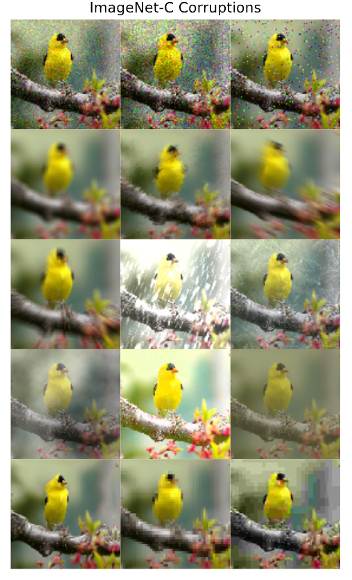Figure 1: Example images from the ImageNet-C dataset

Techniques such as data augmentation, Lipschitz continuation, stable learning, prior learning, and robust network structures have been proposed as ways to improve the robustness of models to address this problem. Among these techniques, data augmentation has received particular attention due to its empirical effectiveness, ease of implementation, low computational overhead, and plug-and-play nature.

There are two major approaches to improving model robustness through data augmentation.

The first is to increase the diversity of the training data by combining multiple random transformations, including AugMix.
AugMix is a method that probabilistically samples a variety of data expansion operations and randomly mixes them to produce a very diverse augmented image, and is a standard data augmentation method (e.g., random inversions and translations), successfully increasing the diversity of the training data.

The second method is to improve the robustness of the model by using adversarial perturbation such as PGD Attack.
In the method using adversarial perturbation, noise is added to the training data to generate images that are difficult for the model to classify, and by learning these images (adversarial learning), the generalization and robustness of the model are successfully improved.

Previous research has focused on leveraging either of these two approaches to improve robustness. Therefore, to improve model robustness, this paper proposes AugMax, an improved method of AugMix that unifies these two approaches (AugMix and adversarial perturbation) in a single framework to improve data diversity and difficulty. AugMax is not only a more powerful data extension and yields heterogeneous input distributions compared to AugMix, but experiments confirm that the proposed method not only achieves state-of-the-art robustness against corruption but also improves robustness against other common distribution shifts.

In the following chapters, I will briefly explain data expansion, AugMix ( the link is to the AugMix article I wrote), and adversarial perturbation as prior knowledge, and then describe the proposed method, experimental details, and results.

## prior knowledge

### What is Data Extension?

Data expansion is a technique to increase the number of data by applying some transformation to the image to simulate the image as shown in Figure 2.
There are countless types of transformations other than those shown in Figure 2, such as Erasing, which fills in a portion of the image, and GaussianBlur, which applies a Gaussian filter.

Models trained with these various extension methods are usually known to be more generalizable than models trained only on the original data, but sometimes they can degrade performance or induce unexpected biases.
Therefore, to improve the generalization rate of a model, effective data extension methods must be manually found based on the domain.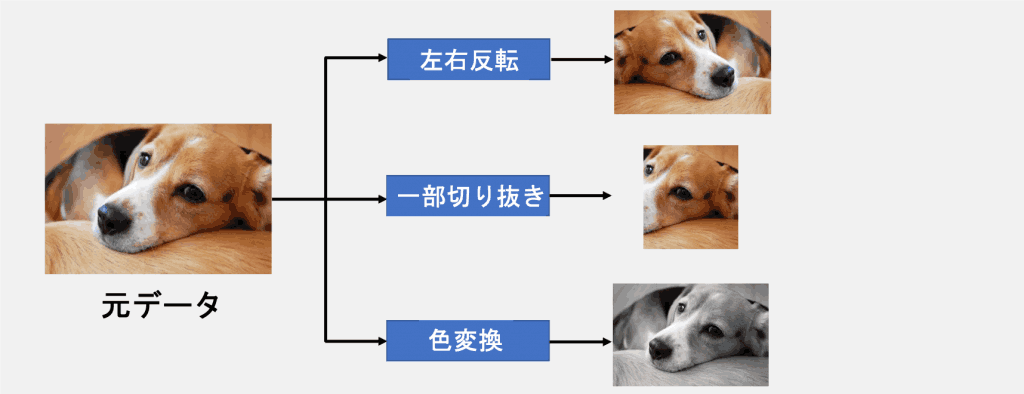Figure 2: Data Extension Overview

### AugMix

A schematic diagram of the AugMix operation is shown in Figure 3.

AugMix is a method for probabilistically sampling a variety of data extension operations from AutoAugment and randomly mixing them to generate a very diverse augmentation image.
This combination is called an Augment Chain, and an Augment Chain consists of a combination of one to three augmentation operations.
AugMix randomly samples $k$ of this Augment Chain (default is $k=3$) and then mixes the sampled (default $k=3$) and synthesizes the sampled Augment Chain using the element-wise convex holomorphic combination.
Finally, the synthesized image is combined with the original image using a "skip connection" to produce an image that preserves image diversity.

If you want to know more detailed parts, please take a look here ( this is the AugMix paper commentary article I authored).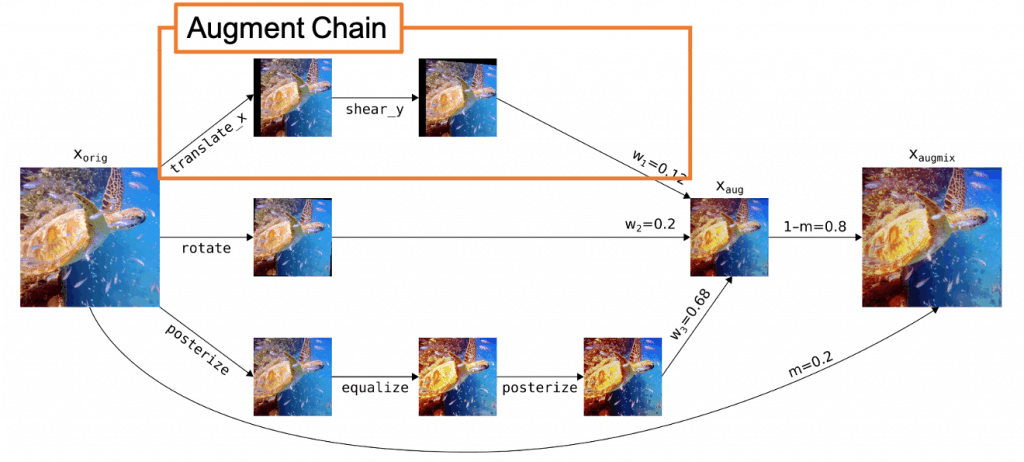Figure 3: Overview of AugMix operation

A hostile perturbation is a hostile perturbation (such as noise) that causes the model to misclassify. The problem with adding hostile perturbations to data is that they cause the model to output incorrect answers with a high confidence level.

A well-known previous study on hostile perturbations is EXPLAINING AND HARNESSING ADVERSARIAL EXAMPLES. The results of this study are shown in Figure 4.

This is because the model judged the image before adding noise (left side of Figure 4) as a panda but judged it as a monkey after adding noise (right side of Figure 4) (it is important to note that the model was not only wrong but also wrong with high confidence). (It is also important to note that the model was not only wrong but also wrong with a high confidence level. (e.g., when using automatic driving, the model may misidentify signs, which may lead to accidents).

Therefore, methods to improve the robustness of models (i.e., accurate classification of images with noise, etc.) have been studied.
In a method using adversarial perturbation, noise is added to the training data to generate images that are difficult for the model to classify, and by learning these images (adversarial learning), the generalization and robustness of the model are successfully improved.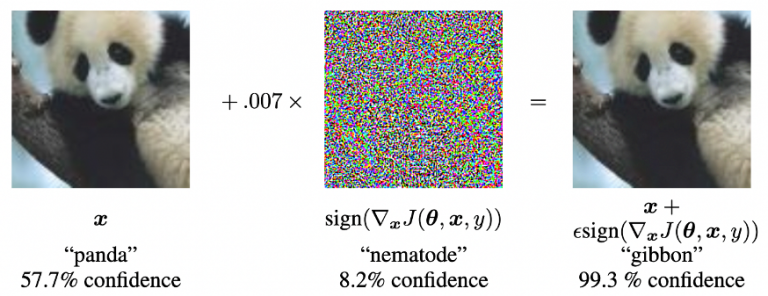Figure 4: Results of previous studies on hostile perturbations

## Proposed Method

We will now describe AugMax, the method proposed in this paper.

AugMax is a data augmentation technique that unifies AugMix and adversarial perturbations into a single framework, thereby increasing the diversity and difficulty of the data and the robustness of the model.

A schematic diagram of AugMax is shown in Figure 5. The left side of Fig. 5 shows the same process as in AugMix, and the right side of Fig. 5 shows the process related to adversarial perturbations, through which the adversarial mixture parameters $w$ and $m$ are updated. This is the main difference between AugMix and the proposed method, AugMax.

The purpose of AugMax is to find the data distribution $\boldsymbol{D}$ of the image $\boldsymbol{x} \in \mathbb{R}^d$ and the label $\boldsymbol{y} \in \mathbb{R}^c$, given $\boldsymbol{D}$, from the image parameterized by $\theta$. Given a data distribution $\boldsymbol{D}$ of $\theta$, we learn a classifier $f$:$\mathbb{R}^d$→$\mathbb{R}^c$, which is parameterized by $\theta$, and as a result, it becomes robust against unknown distributions. So, we initially solve the following equation that minimizes the loss for $\theta$.

$\min _{\boldsymbol{\theta}} \mathbb{E}_{(\boldsymbol{x}, \boldsymbol{y}) \sim \mathcal{D}} \mathcal{L}(f(\boldsymbol{x} ; \boldsymbol{\theta}), \boldsymbol{y})$

where $\mathcal{L}(\cdot,\cdot)$ represents the loss function.

Next, since the image $\boldsymbol{x}^*$ being generated by AugMax on the right side of Figure 5 is generated by learning the adversarial mixture parameters $w$ and $m$, $\boldsymbol{x}^*$ can be expressed as follows.

$\boldsymbol{x}^*=g\left(\boldsymbol{x}_{\text {orig }} ; m^*, \boldsymbol{w}^*\right)$

where $g(\cdot,\cdot)$ denotes the AugMax data expansion function and $\boldsymbol{x}_\text {orig}$ denotes the original image before conversion. Also, the hostile mixing parameters $w$ and $m$ are obtained by solving the following optimization problem.

$m^*, \boldsymbol{w}^*=\underset{m, \boldsymbol{w}}{\arg \max } \mathcal{L}\left(f\left(g\left(\boldsymbol{x}_{\text {orig }} ; m, \boldsymbol{w}\right) ; \theta\right), \boldsymbol{y}\right), \quad$ s.t. $m \in[0,1], \boldsymbol{w} \in[0,1]^b, \boldsymbol{w}^T \mathbf{1}=1$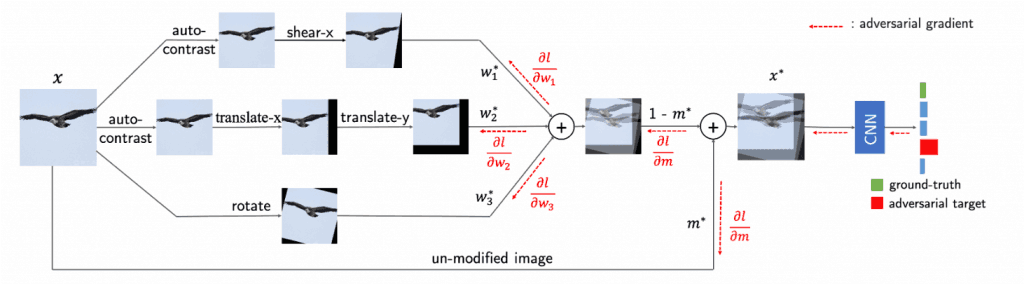Figure 5: Overview diagram of AugMax

## experimental setup

CIFAR10, CIFAR100, ImageNet, and Tiny ImageNet (TIN) were used to evaluate the proposed method.
CIFAR10-C, CIFAR100-C, ImageNet-C, and Tiny ImageNet-C (TIN-C), which were generated by corrupting test set images, were also used as datasets for evaluating the robustness of the model against common natural corruptions.
As model architectures, ResNet18, WRN40-2, and ResNeXt29 are used for the CIFAR dataset, and ResNet18 is used for ImageNet and Tiny ImageNet.

### valuation index

For CIFAR10-C and CIFAR100-C, we evaluated the robustness of the models by defining Robustness accuracy (RA) as the average classification accuracy of 15 types of corruption.
For ImageNet-C and Tiny ImageNet-C, robustness was evaluated using both RA and Mean corruption error (mCE).
Here, mCE refers to the weighted average of the target model corruption errors normalized by the baseline model corruption errors for different types of corruption.

As baseline models, we used AlexNet for the ImageNet experiments and ResNet18, which has been traditionally trained on Tiny ImageNet, for the Tiny ImageNet experiments.
We also used Standard accuracy (SA) for classification accuracy, representing the classification accuracy for the original clean test image.

## Results and Discussion

### CIFAR10-C&CIFAR100-C

For CIFAR10-C and CIFAR100-C, we compared our method with the state-of-the-art method, AugMix, for each architecture.
Figure 6 shows the results for CIFAR10-C and Figure 7 shows the results for CIFAR100-C.
Here, Normal in the table means training with the default standard extension methods (e.g., random inversion and translation).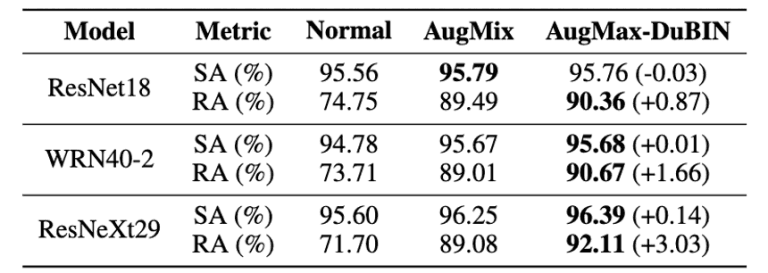Figure 6: CIFAR10-C comparison results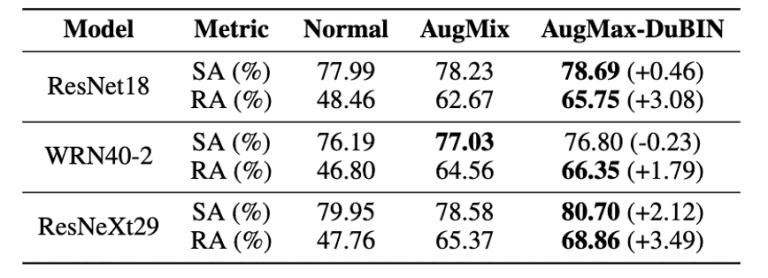Figure 7: CIFAR100-C comparison results

Figures 6 and 7 show that AugMax-DuBIN achieved the best performance on both datasets with different structures.
For example, for CIFAR10-C and CIFAR100-C, we see higher accuracy gains of 3.03% and 3.49%, respectively, compared to AugMix.
In addition, the method was found to be effective for larger models.
Specifically, the largest improvement in robustness was obtained for ResNeXt29, the largest capacity of the three models, in both the CIFAR10 and CIFAR100 experiments.

### ImageNet-C & Tiny ImageNet-C

In prior research, AugMix has been shown to further improve performance on ImageNet-C when combined with other methods such as DeepAugment, and to compare its performance with them, we conducted three comparison experiments on ImageNet-C and Tiny ImageNet-C: (1) AugMix v .s. AugMax, and (2) DeepAugment + AugMix v.s. DeepAugment + AugMax.
Figure 8 shows the results for ImageNet-C and Figure 9 shows the results for Tiny ImageNet-C.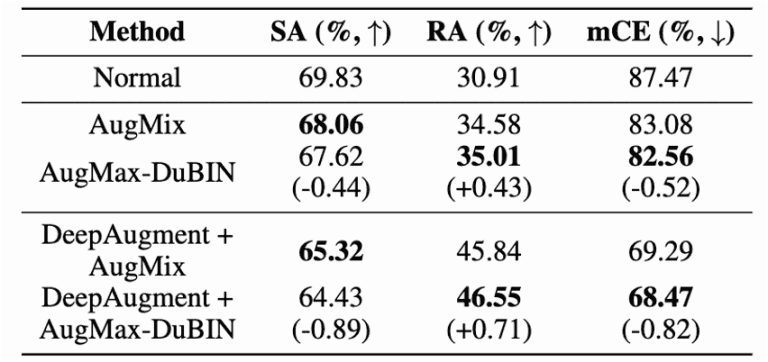Figure 8:ImageNet-C comparison results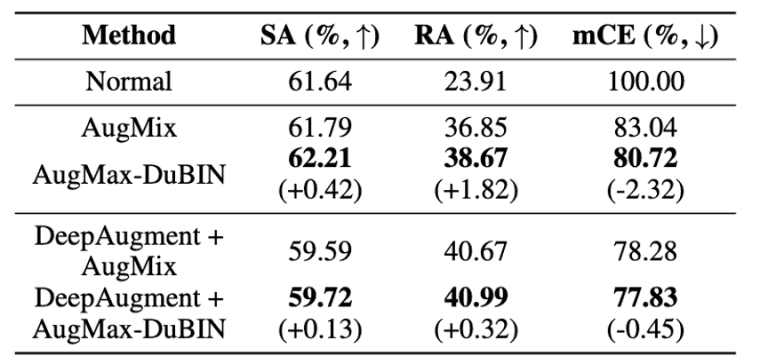Figure 9:Tiny ImageNet-C results

Figure 8 shows that AugMax-DuBIN outperforms AugMix by 0.52% in mCE on ImageNet-C.
Furthermore, the combination of AugMax-DuBIN and DeepAugment outperforms AugMix + DeepAugment by 0.82% in mCE, achieving the latest performance on ImageNet-C.
This shows that AugMax can be used as a more advanced basic building block for model robustness and encourages future research to build other defense methods on top of it.
From Figure 9, Tiny ImageNet-Cconfirms that the proposed method improves mCE by 2.32% compared to AugMix and by 0.45% when combined with DeepAugment.

## summary

This paper proposes "AugMax," a new data expansion strategy that significantly improves robustness by strategically increasing the diversity and difficulty of the data.

The proposed method, AugMax, is a data expansion technique that improves the diversity and difficulty of data and the robustness of the model by combining AugMix, which generates data that does not deviate too much from the original image while maintaining diversity by convex merging multiple transformed images that have undergone data expansion processing, with hostile perturbations data enhancement technology increases data diversity and difficulty and improves model robustness.

To confirm the effectiveness of the proposed method, experiments were conducted to check its performance on CIFAR-10-C, CIFAR100-C, ImageNet-C, andTiny ImageNet, which are datasets with corruption on each of CIFAR-10, CIFAR100, ImageNet, and Tiny ImageNet The performance of the proposed method on CIFAR-10-C, CIFAR100-C, ImageNet-C, and Tiny ImageNet was checked using three indices: Robustness accuracy (RA), Mean corruption error (mCE), and Standard accuracy (SA).
The results show that the proposed method outperforms the state-of-the-art performance in previous studies.

If you have any suggestions for improvement of the content of the article,